# Algebra IPage 1

#### WATCH ALL SLIDES

Slide 1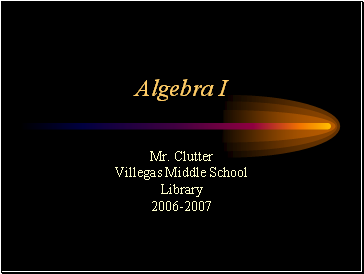Algebra I

Mr. Clutter

Villegas Middle School

Slide 2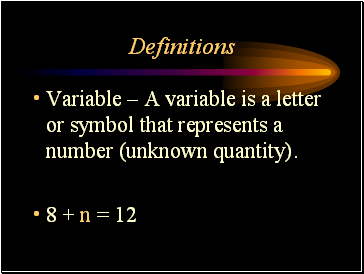## Definitions

Variable – A variable is a letter or symbol that represents a number (unknown quantity).

8 + n = 12

Slide 3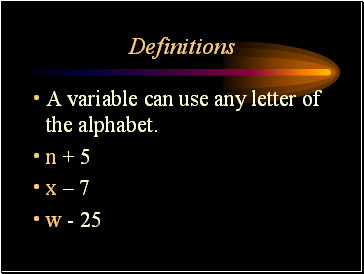Definitions

A variable can use any letter of the alphabet.

n + 5

x – 7

w - 25

Slide 4Definitions

Algebraic expression – a group of numbers, symbols, and variables that express an operation or a series of operations.

m + 8

r – 3

Slide 5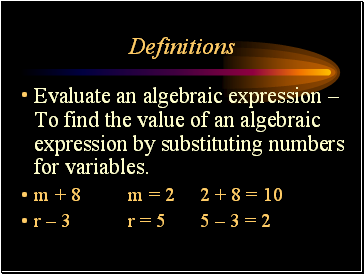Definitions

Evaluate an algebraic expression – To find the value of an algebraic expression by substituting numbers for variables.

m + 8 m = 2 2 + 8 = 10

r – 3 r = 5 5 – 3 = 2

Slide 6Definitions

Simplify – Combine like terms and complete all operations

m = 2

m + 8 + m 2 m + 8

(2 x 2) + 8 4 + 8 = 12

Slide 7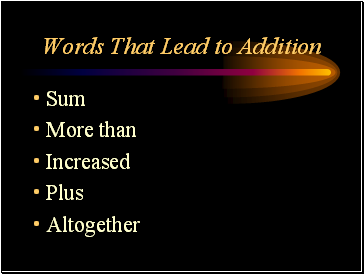Sum

More than

Increased

Plus

Altogether

Slide 8Decreased

Less

Difference

Minus

How many more

Slide 9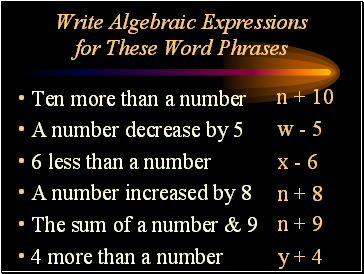## Write Algebraic Expressions for These Word Phrases

Ten more than a number

A number decrease by 5

6 less than a number

A number increased by 8

The sum of a number & 9

4 more than a number

n + 10

w - 5

x - 6

n + 8

n + 9

y + 4

Slide 10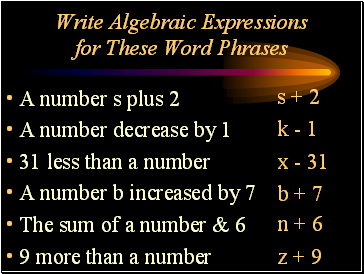Write Algebraic Expressions for These Word Phrases

A number s plus 2

A number decrease by 1

31 less than a number

A number b increased by 7

The sum of a number & 6

9 more than a number

s + 2

k - 1

x - 31

b + 7

n + 6

z + 9

Slide 11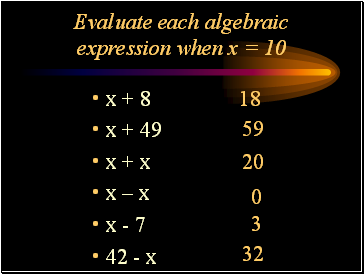## Evaluate each algebraic expression when x = 10

x + 8

x + 49

x + x

x – x

x - 7

42 - x

18

59

20

0

3

32

Slide 12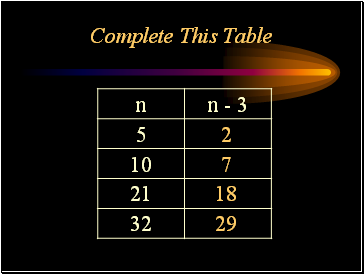Complete This Table

2

7

18

29

Slide 13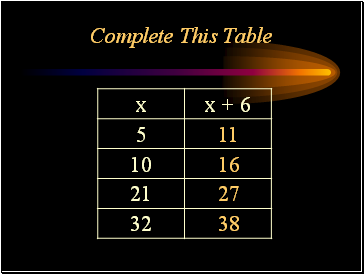Go to page:
1  2